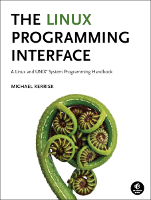This is timers/cpu_multithread_burner.c, an example to accompany the book, The Linux Programming Interface.

This file is not printed in the book; it is a supplementary file for Chapter 23.

In the listing below, the names of Linux system calls and C library functions are hyperlinked to manual pages from the Linux man-pages project, and the names of functions implemented in the book are hyperlinked to the implementations of those functions.• Distribution version

## Function list (Bold in this list means a function is not static)

```/* cpu_multithread_burner.c

loops consuming CPU, and, after each 'period' seconds of consumed CPU,
reports its thread ID, total CPU time consumed, and rate of CPU
consumption since the last report.

For some experiments, it is useful to confine all threads to the
same CPU, using taskset(1). For example:

*/
#define _GNU_SOURCE
#include <sys/syscall.h>
#include <sched.h>
#include <sys/times.h>
#include <time.h>
#include <signal.h>
#include "tlpi_hdr.h"

#if !defined(__GLIBC__) || __GLIBC_MINOR__ < 30
```
```/* glibc 2.30 has a gettid() wrapper */

static pid_t
gettid(void)
{
return syscall(SYS_gettid);
}
#endif

#define NANO 1000000000
```
```static void *
{
float period;       /* Interval (in CPU seconds) for displaying statistics
on consumed CPU time */
char *sarg = arg;
sscanf(sarg, "%f", &period);

struct timespec prev_rt;
if (clock_gettime(CLOCK_REALTIME, &prev_rt) == -1)
errExit("clock_gettime");

long long nloops = 0;
int prev_step = 0;  /* Number of intervals of consumed CPU time calculated
in previous loop iteration */
while (1) {

/* Burn some user-mode CPU time */

int j, k;
for (j = 0, k = 0; j < 1000; j++)
k = j;
if (k >= 0)     /* Use k, to prevent gcc moaning about unused var */
k++;

nloops++;

struct timespec curr_cpu;
errExit("clock_gettime");

/* Calculate current number of intervals of consumed CPU time */

int curr_step = curr_cpu.tv_sec / period +
curr_cpu.tv_nsec / period / NANO;

struct timespec curr_rt;
if (clock_gettime(CLOCK_REALTIME, &curr_rt) == -1)
errExit("clock_gettime");

/* Calculate elapsed real microseconds for current CPU interval */

int elapsed_rt_us = (curr_rt.tv_sec - prev_rt.tv_sec) * 1000000 +
(curr_rt.tv_nsec - prev_rt.tv_nsec) / 1000;

if (curr_step > prev_step) {
printf("[%ld]  %%CPU = %.2f; totCPU = %.3f\n", (long) gettid(),
period / (elapsed_rt_us / 1000000.0) * 100.0,
(float) curr_step * period);
prev_step = curr_step;
prev_rt = curr_rt;
nloops = 0;
}
}

return NULL;
}
```
```int
main(int argc, char *argv[])
{
int j, s;

if (argc < 2 || strcmp(argv, "--help") == 0)
usageErr("%s [period]...\n"
"Creates one thread per argument that reports "
"CPU time each 'period' CPU seconds\n"
"'period' can be a floating-point number\n", argv);

/* Create one thread per command-line argument */

for (j = 1; j < argc; j++) {